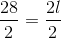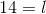ISEE Lower Level Quantitative : Plane Geometry

Example Questions

Example Question #3 : Geometric Measurement: Understanding Concepts Of Area And Relating Area To Multiplication And To Addition

How many square units make up the area of the shape below?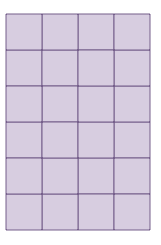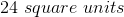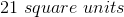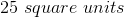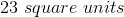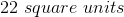Explanation:

The shape is made up of unit squares. We can count the number of squares within the shape to find the area.

There aresquares within the shape.

Example Question #4 : Geometric Measurement: Understanding Concepts Of Area And Relating Area To Multiplication And To Addition

How many square units make up the area of the shape below?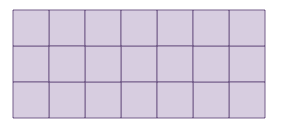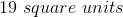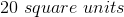Explanation:

The shape is made up of unit squares. We can count the number of squares within the shape to find the area.

There aresquares within the shape.

Example Question #5 : Geometric Measurement: Understanding Concepts Of Area And Relating Area To Multiplication And To Addition

How many square units make up the area of the shape below?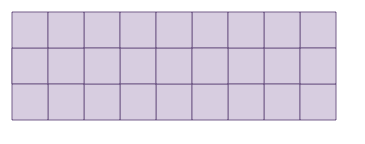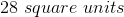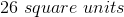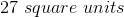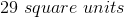Explanation:

The shape is made up of unit squares. We can count the number of squares within the shape to find the area.

There aresquares within the shape.

Example Question #6 : Geometric Measurement: Understanding Concepts Of Area And Relating Area To Multiplication And To Addition

How many square units make up the area of the shape below?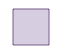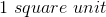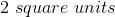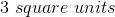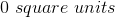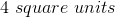Explanation:

The shape is made up of unit squares. We can count the number of squares within the shape to find the area.

There aresquare within the shape.

Example Question #8 : Geometric Measurement: Understanding Concepts Of Area And Relating Area To Multiplication And To Addition

How many square units make up the area of the shape below?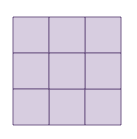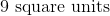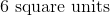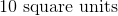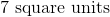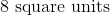Explanation:

The shape is made up of unit squares. We can count the number of squares within the shape to find the area.

There aresquares within the shape.

Example Question #9 : Geometric Measurement: Understanding Concepts Of Area And Relating Area To Multiplication And To Addition

How many square units make up the area of the shape below?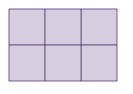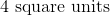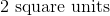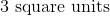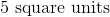Explanation:

The shape is made up of unit squares. We can count the number of squares within the shape to find the area.

There aresquares within the shape.

Example Question #1 : Geometric Measurement: Understanding Concepts Of Area And Relating Area To Multiplication And To Addition

How many square units make up the area of the shape below?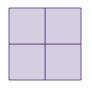Explanation:

The shape is made up of unit squares. We can count the number of squares within the shape to find the area.

There aresquares within the shape.

Example Question #11 : Geometric Measurement: Understanding Concepts Of Area And Relating Area To Multiplication And To Addition

How many square units make up the area of the shape below?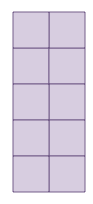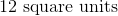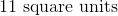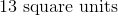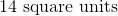Explanation:

The shape is made up of unit squares. We can count the number of squares within the shape to find the area.

There aresquares within the shape.

Example Question #12 : Geometric Measurement: Understanding Concepts Of Area And Relating Area To Multiplication And To Addition

How many square units make up the area of the shape below?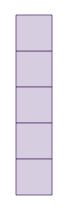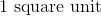Explanation:

The shape is made up of unit squares. We can count the number of squares within the shape to find the area.

There aresquares within the shape.

Example Question #1 : Apply Area And Perimeter Formulas For Rectangles: Ccss.Math.Content.4.Md.A.3

What is the length of a rectangular room with a perimeter of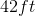and a width of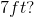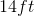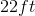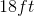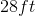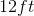Explanation: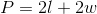We have the perimeter and the width, so we can plug those values into our equation and solve for our unknown.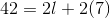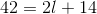Subtractfrom both sides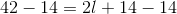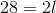Divideby both sides